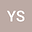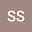Multiple solutions for a class of non-cooperative critical nonlocal equation system with variable exponents
••• Yueqiang Song,
• Shaoyun Shi
Yueqiang Song
Changchun Normal University
Author ProfileShaoyun Shi
Jilin University
Author Profile## Abstract

In this paper, we consider a class of non-cooperative critical nonlocal equation system with variable exponents of the form: $$\left\{ \begin{array}{lll} -(-\Delta)_{p(\cdot,\cdot)}^su - |u|^{p(x)-2}u = F_u(x,u,v) + |u|^{q(x)-2}u, \quad &\mbox{in}\,\,\mathbb{R}^N,\\ (-\Delta)_{p(\cdot,\cdot)}^sv + |v|^{p(x)-2}v = F_v(x,u,v) + |v|^{q(x)-2}u, \quad &\mbox{in}\,\,\mathbb{R}^N,\\ u, v \in W^{s,p(\cdot,\cdot)}(\mathbb{R}^N), \end{array}\right.$$ where $\nabla F = (F_u, F_v)$ is the gradient of a $C^1$-function $F: \mathbb{R}^N\times \mathbb{R}^2 \rightarrow \mathbb{R}^+$ with respect to the variable $(u, v) \in \mathbb{R}^2$. We also assume that$\{x \in \mathbb{R}^N: q(x) = p_s^\ast(x)\} \neq \emptyset$, here $p_s^\ast(x)=Np(x,x)/(N-sp(x,x))$ is the critical Sobolev exponent for variable exponents. With the help of the Limit index theory and the concentration-compactness principles for fractional Sobolev spaces with variable exponents, we establish the existence of infinitely many solutions for the problem under the suitable conditions on the nonlinearity.

#### Peer review status:ACCEPTED

06 Aug 2020Submitted to Mathematical Methods in the Applied Sciences
07 Aug 2020Submission Checks Completed
07 Aug 2020Assigned to Editor
16 Aug 2020Reviewer(s) Assigned
22 Nov 2020Review(s) Completed, Editorial Evaluation Pending
23 Nov 2020Editorial Decision: Revise Minor